﻿ Solving the Laplace’s Equation by the FDM and BEM Using Mixed Boundary Conditions

### Solving the Laplace’s Equation by the FDM and BEM Using Mixed Boundary Conditions

Hassan Ghassemi, Saeid Panahi, Ahmad Reza Kohansal

## Solving the Laplace’s Equation by the FDM and BEM Using Mixed Boundary Conditions

Hassan Ghassemi1,, Saeid Panahi1, Ahmad Reza Kohansal2

1Department of Maritime Engineering, Amirkabir University of Technology, Tehran, Iran

2Department of Shipbuilding, Faculty of Engineering, Persian Gulf University, Bousher, Iran

### Abstract

This paper presents to solve the Laplace’s equation by two methods i.e. the finite difference method (FDM) and the boundary element method (BEM). The body is ellipse and boundary conditions are mixed. In the BEM, the integration domain needs to be discretized into small elements. The boundary integral equation derived using Green’s theorem by applying Green’s identity for any point in the surface. The methods are applied to examine an example of square domain with mixed boundary condition. Both types of numerical models are computed and compared with analytical solution. The results obtained agree perfectly with those obtained from exact solution.

### Cite this article:

• Hassan Ghassemi, Saeid Panahi, Ahmad Reza Kohansal. Solving the Laplace’s Equation by the FDM and BEM Using Mixed Boundary Conditions. American Journal of Applied Mathematics and Statistics. Vol. 4, No. 2, 2016, pp 37-42. http://pubs.sciepub.com/ajams/4/2/2
• Ghassemi, Hassan, Saeid Panahi, and Ahmad Reza Kohansal. "Solving the Laplace’s Equation by the FDM and BEM Using Mixed Boundary Conditions." American Journal of Applied Mathematics and Statistics 4.2 (2016): 37-42.
• Ghassemi, H. , Panahi, S. , & Kohansal, A. R. (2016). Solving the Laplace’s Equation by the FDM and BEM Using Mixed Boundary Conditions. American Journal of Applied Mathematics and Statistics, 4(2), 37-42.
• Ghassemi, Hassan, Saeid Panahi, and Ahmad Reza Kohansal. "Solving the Laplace’s Equation by the FDM and BEM Using Mixed Boundary Conditions." American Journal of Applied Mathematics and Statistics 4, no. 2 (2016): 37-42.

 Import into BibTeX Import into EndNote Import into RefMan Import into RefWorks

1234
Prev Next

### 1. Introduction

Many engineering problems in fluid dynamics, hydrodynamics, elasticity, hydro-acoustics electrostatics, electrodynamics and elasticity are mathematically formulated by partial differential equations (PDEs).

There are many textbooks on BEM to solve the two-dimensional potential problems in fluid dynamics (hydrodynamics and aerodynamics), acoustics, elasticity and structural analysis [1-13].

Solving the Laplace’s equation is an important problem because it may be employed to many engineering problems. Exact solutions of this equation are available and the numerical results may be compared.

Many articles about Laplace’s equation for different problems and various boundary conditions can be found in literature. Here, we present a few of them regarding to our problem. Qian et al solved a Cauchy problem for the Laplace equation in a rectangle . Morales et al studied on the solutions of Laplace’s equation with simple boundary conditions, with consideration to their applications for capacitors with multiple symmetries . Lesnic et al carried out an iterative boundary element method for solving the Cauchy problem for the laplace’s equation . Ren et al studied on analytical evaluation of the BEM singular integrals for 3D Laplace and Stokes flow equations using coordinate transformation . In their paper, by applying a coordinate transformation, the analytical formulas of the singular integrals for 3D Laplace and Stokes flow equations are obtained for arbitrary triangular boundary element with constant elements approximation. The Dirichlet problems of Laplace’s equation in elliptic domains with elliptic holes have studied by Li et al . Lee et al. solved Neumann problems of Laplace’s equation in circular domains with circular holes by methods of field equations. The results show that two kinds of MFEs are effective for solving the Neumann problems and their numerical performances are excellent . The latest work on this kind of equations is Yang’s paper using the Neumann problem of Laplace’s equation in semiconvex domains . In the BEM, solving the integrals are very important and many numerical techniques employed by many researchers. Gao  and Ghassemi & Kohansal  evaluated singular integrals using emerging techniques. They employed the 3D Laplace’s equation to the hydrofoil. Also, singular types of the integrals of order 1/r, 1/r2, 1/r3 using Gauss method evaluated by Jun et al .

The aim of this work is to determine potential in rectangular domain using BEM and FDM using mixed boundary conditions. Comparisons reveal that the methods are efficient and the results are in good agreement with exact solutions.

The paper is arranged hereafter: In section 2, we solve Laplace’s equation in simple geometry by separation of variables. As a matter of illustration of the method, we obtain the potential quantity for this case. In section 3, we use BEM in order to obtain potential at each point of domain. On the other hand, we use FDM in Section 4 to determine the considerable value. Section 5 shows results of two numerical models, BEM and FDM. Also we compare the results with analytical data. Finally, Section 6 contains the conclusions.

### 2. Exact Solution

The Laplace equation and its boundary conditions are defined as follows: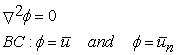(1)

Separation of variables is any of several methods for solving ordinary and partial differential equations, in which algebra allows one to rewrite an equation so that each of two variables occurs on a different side of the equation. It works because it reduces a PDE to ODEs. All the steps of solution can be expressed as follows. In this method we attempt to determine solutions in the product form. Next we place this in Laplace’s equation. Then we claim it is necessary that both sides of the equation must equal to same constant known as the separation constant named Lambda. Now we should do this for any arbitrary constant due to boundary condition. However eventually we will discover that only certain values of Lambda are allowable. Now we obtained the Eigen functions of each variable. The originalis obtained by multiplying together the variables. In summary, we obtained product solutions of the Laplace’s equation satisfying the specific homogenous boundary conditions only corresponding to λ<0. These solutions,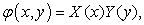haveandwhere we determined from the boundary conditions the allowable values of the separation constant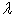,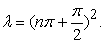Here n is a positive integer.Download asVeiw figureFigures index
Figure 1. Boundary condition

Thus, product solutions of the Laplace’s equation are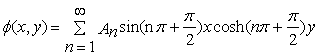where:(2)

So exact solution of equation is obtained as follows(3)

### 3. Boundary Element Method (BEM)

This is a numerical method for solving partial differential equations encountered in mathematical physics and engineering. As the implies method, governing differential equations changes to integral equations that are applied on the surface or boundary. These integrals are numerically integrated over the boundary. The boundary is divided into small elements, as well as other numerical methods ultimately a linear algebraic equation will be obtained which has only one answer. BEM is simply and geometrically applied for any complex shape. In addition, BEM can model areas with sharp changes in variables with accuracy better than the FDM because all approximations limited to the surface. The surface is divided into sections and elements. Shape functions are used to describe the variables and geometries for each element. These shape functions can be linear, quadratic and higher orders. In this way due to the complexity of integrating functions, the analytical integration is not recommended to calculate integrals and numerical integration and Gaussian square method is used instead of it. When the points are close to each other in the calculation of singular integrals or source point p matches the boundary point q, special relationships must be used. Because of the main solution contains orders of 1/r. The total integral is calculated by adding all the integrals on all elements our approach to solve this equation is that the use of fixed element according to the geometry and boundary conditions. Type of elements using the BEM is shown as follows.Download asVeiw figureFigures index
Figure 2. Constant elementDownload asVeiw figureFigures index
Figure 3. Definition sketch of boundary domain

In the BEM for a body of the boundary and domain (as shown in Figure 3), the integral formulation may be expressed as(4)

where:(5)

And G is the green’s function of the Laplace equation.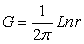(6)

where r distance between the source point (p) and integral element (q). Discretization form of the equation can be represented as follows: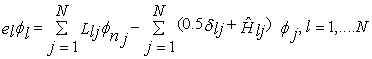(7)

where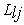andare influence coefficients and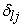is the Kronecker delta.

For the Mixed boundary condition (some boundary Neumann and other Dirichlet) should be put in the Eq (7). The, Eq (7) is arranged to the matrix equation system form, [A]{x}={B}. Finally, it is solved and the unknown variables are obtained.

Furthermore, in the BEM, we solve only for the boundary distribution of the unknown function or one of its derivatives. It is not necessary to compute the requisite function throughout the domain of solution. Once the unknown boundary distribution is available, the solution at any point may be produced by direct evaluation. Thus, the crux of the BEM is the reduction of the dimension of the solution space with respect to physical space by one unit.

### 4. Finite Difference Method (FDM)

FDM is one of the easiest and an oldest numerical method because of its simplicity is commonly used by engineers. However, due to the inability of this method in spatial discretization of non-rectangular and complex geometries, its usage is limited to relatively simple and rectangular geometry issues. In FDM Taylor series expansion and equations such as these are used. Derivatives and equations approximate and directly replace with various terms in the equations. Finite difference estimates are discrete model of continuous finite difference operators. They are used to provide a discrete model of a partial differential equation. Finite differences associated with the derivative of a function estimate at a point such as X0 obtained by using the function values in the vicinity of the point. These estimates have been usually formed of function values in a certain number of points that have been placed at the same distance apart. Estimates of finite difference can be divided to smaller categories of backward, forward and central. For this article according to the Laplace’s equation central three-point discretization for second order derivatives were used in the following equations: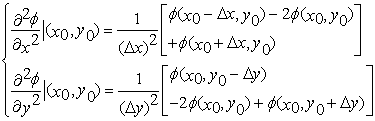(8)

whereBy substituting x in Laplace’s equation, discretized equation can be obtained as follows: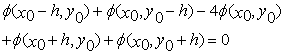(9)

After discretization, boundary conditions must be applied. The boundary condition on Dirichlet and Neumann type is applied directly in the discrete equation. Boundary derivatives can be approximated with finite difference and placed it in the system of linear equations. One thing that is important is that truncation error finite difference estimations for boundary derivative should be equal to finite difference truncation error for differential equation, because of its high accuracy in validation. In Figure 4, geometry of equation by considering mesh generation with 32 elements has been shown.Download asVeiw figureFigures index
Figure 4. Domain discretization in FDM with mixed boundary conditions

### 5. Numerical Results

In the BEM a simple body with 24 elements is considered. The body is discretized into constant elements. The geometry of the 24 nodes of the elements on the body is shown in the Figure 5.

Comparison of the numerical and analytical results are shown through Tables 1and 2 when the field point is located on the middle of each element. The approach of this paper is based on considering equal elements on boundary for BEM and FDM to compare these numerical methods with each other in same level of accuracy. The results are compared in terms of relative error, defined by(10)

whereandare the approximate and exact values under consideration.Download asVeiw figureFigures index
Figure 5. Boundary discretization in BEM with mixed boundary conditions

In order to estimate the accuracy of methods for evaluating potential in internal nodes that appearing in (1), the rectangle inside the domain should be considered, (Figure 6). Side 1 tends to points with x=0.25 and variable y, 2 are points with x=0.75, 3 are points with y=0.25 and variable x, and side 4 hint points with y=1.75. Comparisons of the numerical and analytical calculations for the potential in each side of rectangle are shown through Figure 7-Figure10.

#### Table 1. Comparison of the analytical and BEM

The relative error is given in the Table 1 that is less than 1e-03 except for the (0.75, 0.75) point in BEM. As shown in the Table 2, the maximum error is about 0.22 where the field point is on the (0.25, 0.25) point. Similar trends are obtained when the field points are located far from upper side of the domain, relative error gradually increases. It is shown that when the solutions have more error the results of the final calculation on the elements may not obtained precisely.

#### Table 2 Comparison of the analytical and FDMDownload asVeiw figureFigures index
Figure 6. Internal nodesDownload asVeiw figureFigures index
Figure 7. Comparison of the numerical and analytical calculations for the potential distribution side 1Download asVeiw figureFigures index
Figure 8. Comparison of the numerical and analytical calculations for the potential distribution side 2Download asVeiw figureFigures index
Figure 9. Comparison of the numerical and analytical calculations for the potential distribution side 3Download asVeiw figureFigures index
Figure 10. Comparison of the numerical and analytical calculations for the potential distribution side 4

### 6. Conclusions

In this paper, a two dimensional numerical model using the BEM was introduced to predict the potential characteristics of various point of domain. In addition, the FDM applied to compare with. The numerical results of the potential distributions were compared against exact data and shown to be a good agreement. As a result of the present work, the following conclusions can be written:

• A comparison of the potential distributions for the various points of geometry shows satisfactory results compared with those exact and numerical data.

• Potential distribution exerted on domain can be successfully predicted through the methods presented in this paper.

• For linear and elliptic problems the BEM is vastly superior in both efficiency and accuracy.

• Alternative methods require discretizing the whole of the solution domain, and this considerably raises the cost of the computation.

• Problems that can be solved on a laptop computer using the BEM may require the use of a supercomputer by FDM for the same level of accuracy.

Greater understanding of the other quantities like velocity, pressure and flow distribution in various geometries is required. This is a research areas that the authors intend to pursue in more detail in the future

### References

  Katsikadelis, J. T., Boundary element, theory and application, Elsevier publication, 2002.In article  Sauter S.A., Schwab C., “Boundary Element Method,” Springer-Verlag Berlin, 2011.In article View Article  Selvadurai A.P.S., “Partial Differential Equations in Mechanics 1: Fundamentals, Laplace’s Equation, Diffusion Equation, Wave Equation,” Springer-Verlag Berlin Heidelberg, First edition, 2000.In article  Gilbarg D., Trudinger N.S., “Elliptic Partial Differential Equations of Second Order,” Springer-Verlag Berlin Heidelberg New York, 2001.In article  Pozrikidis C., “A Practical Guide to Boundary Element Methods with the Software Library BEMLIB,” Chapman & Hall/CRC, 2002.In article View Article  Beer G., Smith I., Duenser C., “The Boundary Element Method with Programming,” Springer Wien Newyork, 2008.In article  Aliabadi M. H., Wen P. H., “Boundary Element Methods in Engineering and Sciences,” Imperial College Press, 2011.In article  Masataka T., Thomas A. Cruse, “Boundary Element Methods in Applied Mechanics,” Pergamon press, 1988.In article PubMed  Heinz Antes, “A Short Course on Boundary Element Methods,” lecture notes, Technische Universitat Braunschweig, 2010.In article  Ramachandran P. A., “Boundary Element Methods in Transport Phenomena,” Washington University, 1994.In article  Carrier G.F., Pearson C.E., “Partial Differential Equations: Theory and Technique”, 2nd edition, New York Academic press, 1988.In article  Banerjee P.K., “The Boundary Element Methods in Engineering,” McGraw-Hill, London, 1994.In article  Ghassemi, H., Bakhtiari M., Boundary element methods, Amirkabir University of Technology publication, 2016. (in Persian).In article  Qian Z., Fu C.L., Li Z.P., “Two regularization methods for a Cauchy problem for the Laplace equation,” Mathematical Analysis and Applications, May 2007.In article  Morales M., Rodolfo Diaz R.A., Herrera W. J., “Solutions of Laplace’s equation with simple boundary conditions, and their applications for capacitors with multiple symmetries,” Journal of electrostatics, 78 (2015) 31-45.In article View Article  Lesnic D., Elliott L. and Ingham D.B., “An iterative boundary element method for solving numerically the Cauchy problem for the Laplace equation,” Engineering Analysis with Boundary Elements, Vol-20, Issue 2, September 1997.In article  Ren Q., Chan C.L., “Analytical evaluation of the BEM singular integrals for 3D Laplace and Stokes flow equations using coordinate transformation,” Engineering Analysis with Boundary Elemens, Vol-53, pp1-8, April 2015.In article  Li Z.C., Zhang L.P., Wei Y., Lee M.G., Chiang J.Y., “Boundary methods for Dirichlet problems of Laplace’s equation in elliptic domains with elliptic holes,” Engineering Analysis with Boundary Elements, Vol-61, December 2015.In article  Lee M.G., Li Z.C., Huang H.T., Chiang J.Y., “Neumann problems of Laplace’s equation in circular domains with circular holes by methods of field equations,” Engineering Analysis with Boundary Elements, Vol-51, Feb 2015.In article  Yang S., “The Neumann problem of Laplace’s equation in semionvex domains,” Nonlinear Analysis: Theory, Methods & Applications, Vol-133, March 2016.In article  Gao X.W., “Evaluation of regular and singular domain integrals with boundary-only discretization-theory and Fortran code,” Journal of Computational and Applied Mathematics, 175 (2005) 265-290.In article View Article  Ghassemi H., Kohansal A.R., “Numerical evaluation of various levels of singular integrals arising in BEM and its application in hydrofoil analysis”, Applied Mathematics and Computation. 213 (2009) 277-289.In article View Article  Jun L., Beer G., Meek J., “Efficient evaluation of integrals of order 1/r, 1/r2, 1/r3 using Gauss method,” Eng. Anal. 2 (1985) 118-123.In article View Article